[BACK]Computer Modeling in Engineering & SciencesDOI: 10.32604/cmes.2021.015685

ARTICLE

1College of Mechanical and Electrical Engineering, Qingdao University, Qingdao, 266071, China
2Power Integration and Energy Storage System Engineering Technology Center, Qingdao University, Qingdao, 266071, China
*Corresponding Author: Qinghai Zhao. Email: zqhbit@163.com
Received: 04 January 2021; Accepted: 24 February 2021

Abstract: This paper presents a robust topology optimization design approach for multi-material functional graded structures under periodic constraint with load uncertainties. To characterize the random-field uncertainties with a reduced set of random variables, the Karhunen-Loève (K-L) expansion is adopted. The sparse grid numerical integration method is employed to transform the robust topology optimization into a weighted summation of series of deterministic topology optimization. Under dividing the design domain, the volume fraction of each preset gradient layer is extracted. Based on the ordered solid isotropic microstructure with penalization (Ordered-SIMP), a functionally graded multi-material interpolation model is formulated by individually optimizing each preset gradient layer. The periodic constraint setting of the gradient layer is achieved by redistributing the average element compliance in sub-regions. Then, the method of moving asymptotes (MMA) is introduced to iteratively update the design variables. Several numerical examples are presented to verify the validity and applicability of the proposed method. The results demonstrate that the periodic functionally graded multi-material topology can be obtained under different numbers of sub-regions, and robust design structures are more stable than that indicated by the deterministic results.

Keywords: Multi-material; topology optimization; robust design; periodic functional gradient; sparse grid method

1  Introduction

Functionally graded structure, known as non-uniform structure, has extensive application prospects in the fields of aerospace, aircraft design, communication and electronics, etc., due to excellent performances such as vibration absorption, heat insulation and noise reduction.

In recent years, there is a trend to optimize the design of functionally graded structures using topology optimization methods [1,2] to achieve specific performances. Paulino et al.  firstly discussed the effectiveness of the functionally graded structure topology optimization design using the criterion method. Since then, great achievements have been made in the study of topology optimization for functionally graded structures design. Xia et al.  achieved the parallel optimization design of material properties and material distribution of functionally graded structures. Huang et al.  employed the bi-directional evolutionary structural optimization (BESO) approach to obtain various anisotropic microstructures of porous materials and composite materials. Recently, a systematic multi-phase infill design method  is proposed to generate graded multi-material infill structures. According to the above introduction, existing topological optimization methods of functionally gradient structures generally ignore the influence of uncertain factors such as material uncertainty [7,8], load uncertainty [9,10], geometric size and boundary uncertainty [11,12], resulting in structural instability and unreliability after optimization. In this context, structural topology optimization considering uncertainties tends to make optimized topologies more stable and reliable [13,14].

Currently, topology optimization under loading uncertainties is generally classified into two categories, namely reliability-based topology optimization (RBTO) [15,16] and robust topology optimization (RTO) [17,18]. RBTO focuses on safety and aims to accurately provide structural safety measures, while RTO attaches importance to structural stability, which reduces the sensitivity of structural performance. Ben-Tal et al.  firstly employed the semidefinite programming to solve the RTO problem of truss structures. Compared with the discrete structure, the RTO problem of continuum structure is more practical and has become one of the research hotspots. Amir et al.  proposed a reanalysis method to solve RTO problem, aiming to improve the computational efficiency of RTO algorithm. Wu et al.  explored the RTO method for uncertainties of external load direction based on interval variables. Moreover, Zhang et al.  investigated a robust topology optimization method for designing the microstructures of PnCs by considering random field material properties. However, the above researches mainly focus on RTO of single material structures. The emergence of 3D additive printing technology makes the manufacturing and processing of multi-material structure possible. Recently, Kang et al.  proposed a robust shape and topology optimization method considering the uncertainties with the interface of multi-material structures. Chan et al.  presented a density-based RTO for meso- or macroscale multi-material lattice structures under any combination of material and load uncertainties. Zheng et al.  proposed an effective method for designing multi-material robust structures, aiming at minimizing the compliance of linear elastic structures. Romero et al.  performed the multi-material robust topology optimization problem by minimizing the topology derivative constructor of the functional to achieve the nucleation and evolution of the level set.

This paper optimizes the topology of the gradient layer with the mean and standard deviation weights of the structural compliance as the objective function and the volume fraction as the constraint to identify the optimal multi-material layout scheme. Meanwhile, considering the application in practical engineering, periodic constraints are applied in the macrostructure topology optimization model for easy manufacturing and assembly. A robust topology optimization design method for multi-material functionally graded structures with periodic constraints is proposed, which applies the Ordered-SIMP method to characterize the multi-material interpolation model. In terms of load random field distribution, the K-L expansion is employed to transform the random field into a finite number of unrelated load random variables, and the sparse grid numerical integration method is adopted to transform the RTO into a set of multi-weighted deterministic topology optimization (DTO).

The rest of the paper is organized as follows. Section 2 introduces the periodic multi-material functionally graded structure. Section 3 gives a brief introduction of multi-material structure interpolation model. The uncertainties in the characterization of the loading uncertainty with random fields are described in Section 4. Section 5 presents the establishment development and solution procedure of the proposed RTO formulation. Several numerical examples are given in Section 6 to verify the proposed method. Section 7 summarizes the remarkable conclusions.

The macro design domain of a periodic multi-material functionally graded structure is divided into multiple gradient layers (Ω1,,Ωz,,Ωn), where each gradient layer consists of periodic material microstructures. Firstly, given the initial conditions of the structural Dirichlet boundary ΓD, Neumann boundary ΓN and external load, the topology optimization design of the macrostructure obtain the optimal results of the material density at each gradient layer. Furthermore, the macroscopic structure of the gradient layer and the microstructure of the material are optimized to obtain the optimal distribution of the microstructure of the periodic functionally graded material. Periodic multi-material functionally graded structure is depicted in Fig. 1.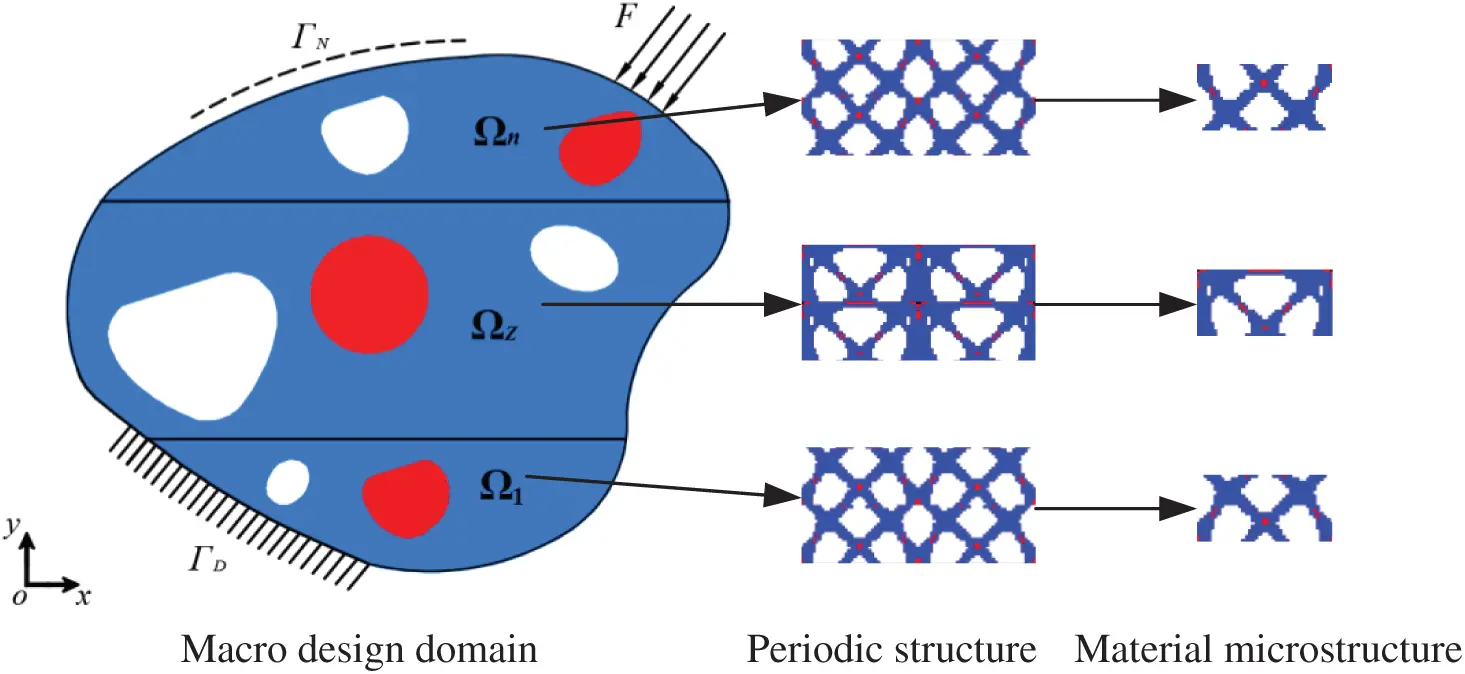Figure 1: Periodic multi-material functionally graded structure

3  Multi-Material Interpolation Model Based on Ordered-SIMP

The SIMP method introduces cell design variables that vary continuously in the interval of 0–1  to establish a nonlinear functional relationship between material properties and design variables.

In terms of the design of multiple materials structure, the elastic modulus and density of each material are firstly normalized, and the material properties are transformed into dimensionless relative values

{EmN=Em0/EmaxρmN=ρm0/ρmax(m=1,2,,M)(1)

where EmN and ρmN represent the elastic modulus and density of the material m after normalization, E0m and ρm0 denote the elastic modulus and density of the solid material m, Emax and ρmax are the elastic modulus and density of all materials, respectively, M is the total number of materials.

The multiple material interpolation model based on the ordered-SIMP method  can be expressed as

Em(xe)=Pm(xe)β+Omxe[ρmN,ρm+1N](2)

where xe denotes the relative density of element e, which is the design variable, β is the penalty factor, Em is the elastic modulus after multi-material interpolation, ρm+1N represents the density of the material m + 1 after normalization. Pm and Om respectively represent scale coefficient and translation coefficient, which can be formulated as

{Pm=EmN-Em+1N(ρmN)β-(ρm+1N)βOm=EmN-Pm(ρmN)(3)

where Em+1N represents the elastic modulus of the normalized material m + 1. Fig. 2 shows the interpolation curve of three-angle materials based on the Ordered-SIMP method (ρ1N=0.4, ρ2N=0.7, ρ3N=1; E1N=0.2, E2N=0.8, E3N=1.0), where, — represents β=0, - - - represents β=3, -- represents β=9. It can be seen from the figure that the penalty effect increased significantly with the increase of β. In particular, the interpolation model is linear and has no penalty effect when β=1.0.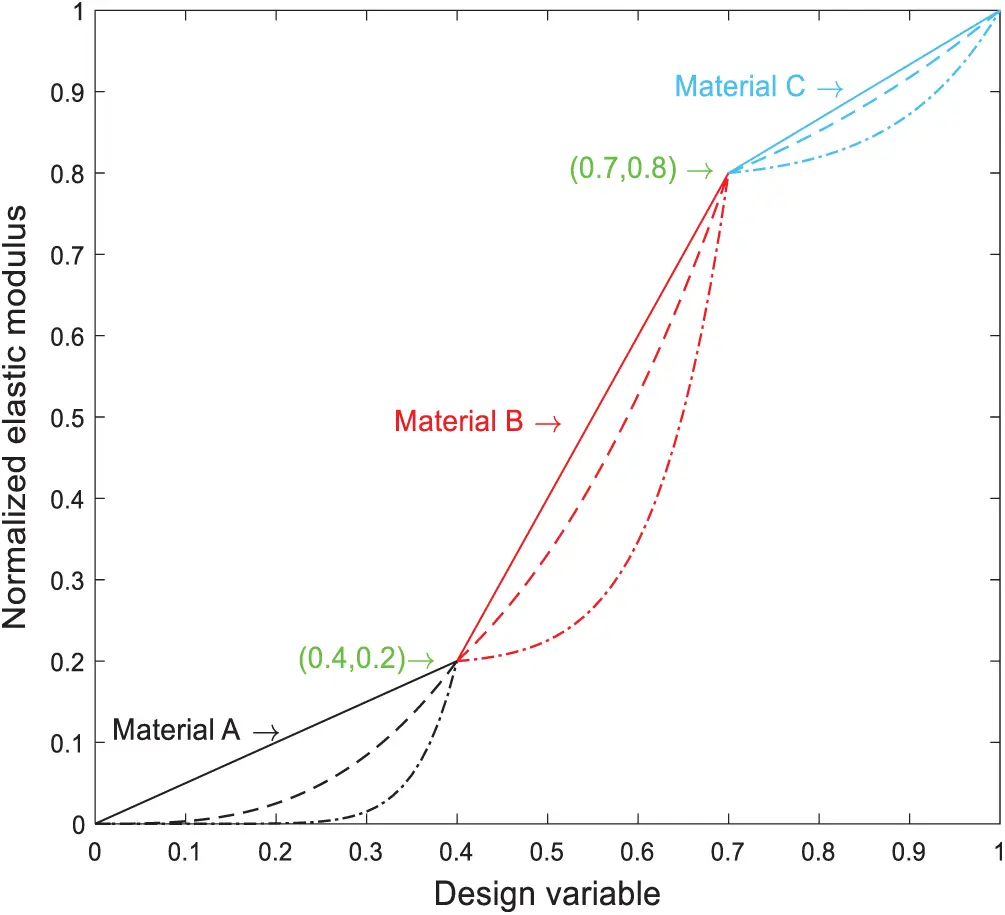Figure 2: Three materials interpolation model based on Ordered-SIMP

4  Discretization of Random Field under Load Uncertainties

The load uncertainties can be characterized by random field or random variable. For distributed loads with spatial correlation, the commonly used discrete and simulation methods include the extended optimal linear estimation method  and the K-L expansion [32,33]. This paper uses the K-L expansion to transform the random field into the cumulative sum of a finite number of uncorrelated random variables under corresponding weights . The K-L expansion of the random field can be described as

ζ(ϑ,θ)=e(ϑ)+i=1λiφi(ϑ)μi(θ)(4)

where ζ(ϑ,θ) denotes the two-dimensional random load field in the continuous space domain D, ϑ is the space coordinate, defined as ϑ=(ϑ1,ϑ2); θ is the random coordinate, e(ϑ) is the mean value, λi and ϕi are the i-th eigenvalue and orthogonal eigenvector of the random field covariance C(ϑ1,ϑ2) respectively. Random field covariance C(ϑ1,ϑ2) satisfies Fredholm integral equation

DC(ϑ1,ϑ2)φi(ϑ1)dϑ1=λiφi(ϑ2)(5)

where μi(θ) denotes uncorrelated random variable, which satisfies the following conditions

E[μi(θ)]=0;E[μi(θ),μj(θ)]=δij(6)

where δij is Kronecker-delta function, δij=1 for i = j, and 0 for others. Independent orthogonal random variables are defined as

μi(θ)=1λiD[ζ(ϑ,θ)-e(ϑ)]φi(ϑ)dϑ(7)

When the K-L expansion is applied to the discretization of random field ζ(ϑ,θ), a d-dimensional random vector ζ(ϑ) is define, whose elements are mapped to d observations in ζ(ϑ,θ). Then the K-L expansion of the load random field can be given by

ζ(θ)=e+i=1λiφiμi(θ)(8)

where e is the mean value of the random field at d observation points; λi and ϕi are the i-th order eigenvalue and orthogonal eigenfunction of the correlation matrix C, which can be solved by the following formula 

Cφi=λiφi(9)

where the correlation matrix C is defined as

C=[C(ϑ1,ϑ1)C(ϑ1,ϑ2)C(ϑ1,ϑd)C(ϑ2,ϑ1)C(ϑ2,ϑ2)C(ϑ2,ϑd)C(ϑd,ϑ1)C(ϑd,ϑ2)C(ϑd,ϑd)](10)

In practical problems, the main probability characteristics of a stochastic process are usually approximated by the random variables corresponding to the first few eigenvalues descending from the maximum eigenvalue. Taking the first M items and letting it as Md, and the determination of the random field using the reduced dimensional K-L expansion can be expressed as follows:

s=i=1Mλii=1dλi(11)

when s is close enough to 1, the random field can be effectively characterized by dimensionality reduction K-L expansion [36,37].

5  Robust Topology Optimization Model of Multi-Material Functionally Graded Structure

5.1 Robust Topology Optimization Model

The purpose of robust topology optimization design is to reduce the sensitivity of performance function to uncertain random variables while optimizing the objective function, so as to minimize the variance of performance index fluctuation . The difference between the deterministic optimal solution and the robust optimal solution is depicted in Fig. 3. It can be seen that the optimal performance of the robust design is worse than the former, but its performance index is less volatile and more superior to a certain extent. Therefore, robust topology optimization design, as a typical multi-objective optimization design problem, is usually achieved by weighting the expectation and standard deviation of the performance function into the objective function.

According to the mathematical description of uncertain variables, robust topology optimization design can be divided into non-probabilistic and probabilistic models . Probabilistic models can be used to describe the characteristics of most uncertain factors. Based on probability theory and mathematical statistics, typical indicators for measuring the robustness of structural performance are termed as mean and standard deviation. The mathematical model for robust topology optimization of multi-material structures is established as follows:

findxe={x1,x2,,xNe}TRminJ=wμc(xe,ζ)+τσc(xe,ζ)s.t.V(x)=e=1NexevefV0K(xe,ζ)U(xe,ζ)=F(ζ)0<xminxexmax1(12)

where J is the objective function, w and τ are the weighting coefficients, and the summation of the weighting coefficients equals to 1, xe represents the deterministic variable, namely multi-material structure topology optimization design variable, uncertainty variable ζ obeys a certain probability distribution, K, U and F are the stiffness matrix, displacement vector and structural load, respectively, ve is the volume of the element; V0 and f are the ratio of the total volume and the volume fraction of the design area, Ne is the total number of element, xmax and xmin are the upper and lower limits of the design variables, respectively, μc(xe,ζ) and σc(xe,ζ) are the mean and standard deviation of the structural compliance, given by 

μc(xe,ζ)=E[c(xe,ζ)]=Dc(ζ)p(ζ)dζ (13)

σc(xe,ζ)=E{[c(xe,ζ)-μc(xe,ζ)]2}=D[c(ζ)-μc(xe,ζ)]2p(ζ)dζ (14)

where p(ζ) denotes the joint probability density function of ζ, the structural compliance c(xe,ζ) can be expressed as

c(xe,ζ)=12U(xe,ζ)TK(xe,ζ)U(xe,ζ)(15)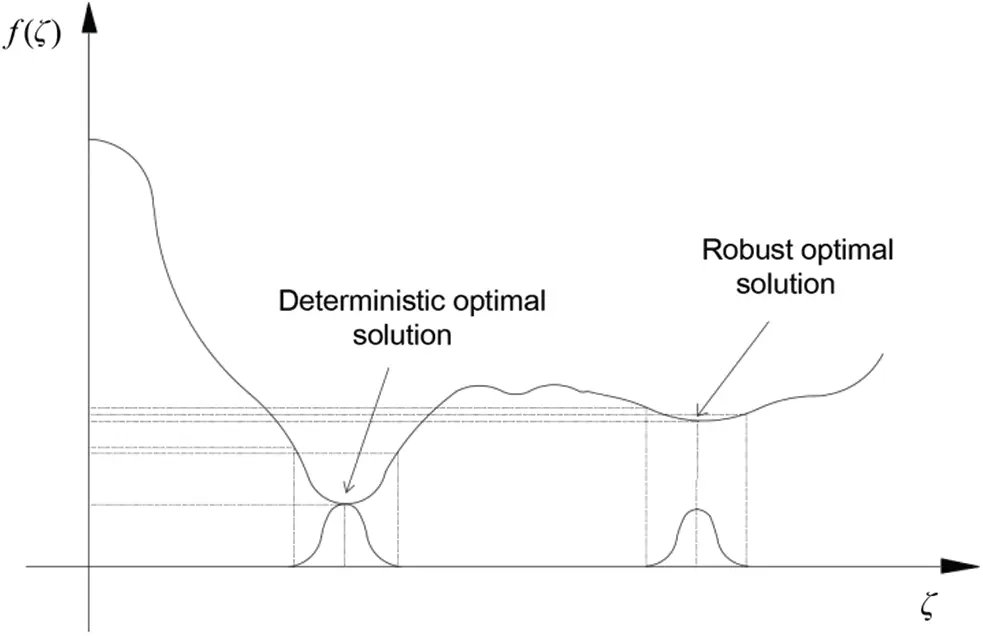Figure 3: Deterministic optimal solution and robust optimal solution

5.2 Robust Topology Optimization Model of Functionally Graded Structure

In order to calculate the overall layout of a functionally graded structure [41,42] under volume constraints. The volume fraction of each preset gradient layer in the optimal topological configuration is extracted from the multi-material structure topology optimization model. The two-dimensional rectangular design domain is divided into n gradient layers: Layer 1,, Layer z,, Layer n, where n is the total number of divided layers, and the layer-wise design is shown in Fig. 4.

Then, each gradient layer is individually designed for optimization. The topology optimization model of the functionally graded structure design can be defined as

findxe,z(e=1,2,,N;z=1,2,,n)minJ=wμc(xe,z,ζi)+τσc(xe,z,ζi)s.t.V(x)=e=1Nxe,zve,zfzVzK(xe,z,ζi)U(xe,z,ζi)=F(ζi)0<xminxe,zxmax1(16)

where N represents the number of element in the gradient layer, xe,z is the design variable of the z gradient layer element e, ve,z is the element volume of the z gradient layer element e, Vz and fz are the volume and the volume fraction of the z gradient layer.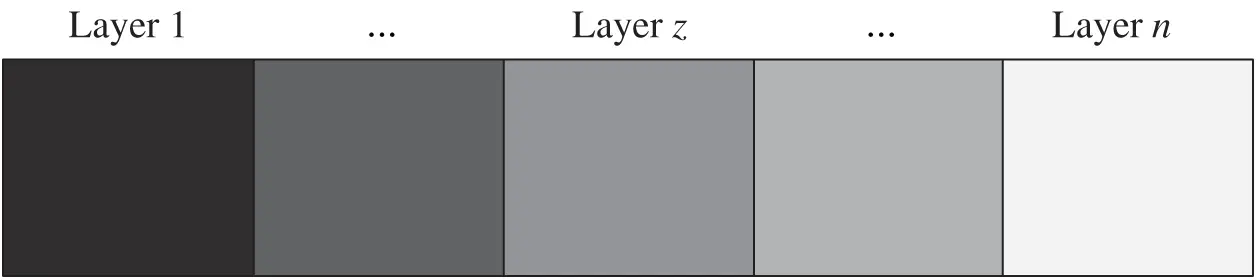Figure 4: Layer-wise design

The optimization goal of this paper is the weighted sum of the mean and standard deviation of structural compliance. Among them, the sensitivity of the mean and standard deviation relative to the design variable xe,z can be given by

μc(xe,z,ζi)xe,z=E[c(xe,z,ζi)xe,z] (17)

σc(xe,z,ζi)xe,z=1σcE{[c(xe,z,ζi)-μc(xe,z,ζi)][σc(xe,z,ζi)xe,z-μc(xe,z,ζi)xe,z]} (18)

5.3 Sparse Grid Numerical Integration Method

In order to solve the objective function of the robust topology optimization design, a sparse grid method based on the Smolyak criterion  is employed. A basic idea is to construct a multidimensional product formulation using a special tensor product operation of one-dimensional configuration points as a linear combination. The advantage of this method is that the number of configuration points is limited, and the nodes that contribute less to the calculation accuracy are automatically deleted, so the sparse grid is applied to the robust design of statistical moment estimation. Based on the nested hierarchy principle, the one-dimensional interlayer difference is defined as

Δk1(c)=(ψk1-ψk-11)(c)(19)

where k represents the dimension, when k = 0, ψk1=0, for the performance function c with d-dimensional loading random variables, the sparse grid numerical integration format with l-level (l1) precision is constructed as

ψld(c)=|k|l+d(Δk11Δkd1)(c)=l+1|k|l+d(-1)l+d-|k|(d-1l+d-|k|)(ψk11ψkd1)(c)(20)

where denotes the tensor product operator, |k| is the multidimensional index accumulation, which satisfies |k|=k1+k2++kd. The corresponding set of sparse grid configuration points is defined as

Ûld=l+ 1|k|l+d(Uk11Uk21Ukd1)(21)

where Ukd1 is the configuration points of the d-th dimension. According to Smolyak criterion, the corresponding d-th configuration points in Ûld is defined as

ζi=(ζk1e1,,ζkded)(22)

The corresponding weights are determined as

we=(-1)l+d-|k|(d-1l+d-|k|)(wk1e1,,wkded)(23)

Thus, the configuration points are given as

ald=l+ 1|k|l+d(mk11mkd1) (24)

By adjusting the level accuracy l value, the sparse grid integration accuracy is improved. Based on the Newton-Cotes integration rule, the Causs–Chebyshev  sparse grid HT[-1,1] is constructed, and the one-dimensional configuration points are as follows:

uke={- cos(πe-1mk-1)fore=1,2,,mk,ifmk>10ifmk=1(25)

The sequence of figuration points is

mk={1ifk=12k-1+1if k>1(26)

Then, the corresponding weights are expressed as follows:

wke={1mk(mk-2)fore=1,mk,if mk>12(mk-1)(1-cosπ(e-1)mk(mk-2)-2j=1(mk-3)/214j2-1 cos2πj(e-1)mk-1)fore=2,,mk-1,ifmk>12ifmk=1(27)

The sparse grid numerical integration method is employed to solve the mean and the standard deviation of the robust topology optimization design. The expression of mean and standard deviation can be modified as

μc=E[c(xe,z,ζ)]=c(ζ)p(ζ)dζe=1aldwec(ζe) (28)

σc=E{[c(xe,z,ζ)-μc(x,ζ)]2}={[c(ζ)-μc]2p(ζ)d(ζ)}1/2{e=1ald we[c(ζe)-μc]2}1/2 (29)

According to Eqs. (17) and (18), the derivative of the optimization target J with respect to the density xe,z is

Jxe,z=μcxe,z+σcxe,ze=1ald wec(ζi)xe,z+1σce=1ald we[c(ζi)-μc][c(ζi)xe,z-μcxe,z](30)

The commonly used optimization solution algorithms include mathematical programming method and optimization criterion method. In the iterative process, MMA  has better applicability for problems with complex objectives and multi-constrained topology optimization. In this paper, MMA method is introduced to solve the topology optimization problem of multi-material functional graded structure. And the PDE filtering method based on Helmholts equation is adopted.

5.4 Periodic Setting

In order to obtain the periodic functionally graded structure, the elements of the different substructures of each gradient layer at the same position have the same material properties. The periodic layer-wise design is shown in Fig. 5. Each gradient layer is divided into Mxj×Myj identical sub-regions, where Mxj and Myj represent the number of sub-regions in the x and y axes directions of the j-th gradient layer, respectively. The mathematical model of periodic structure can be described as

x1e=x2e==xMxj×Myje,e=1,2,,N(31)

By reallocating the average element compliance in sub-regions, the periodic constraint setting of the gradient layer is achieved.

(Cp¯)1i=(Cp¯)2i==(Cp¯)Mxz×Myzi=1Mxz×Myze=1Mxz×Myz(Cp¯)ei,i=1,2,,N(32)

At this point, the sensitivity of elements at the same position is equal to that of different substructures to achieve periodic geometric constraints.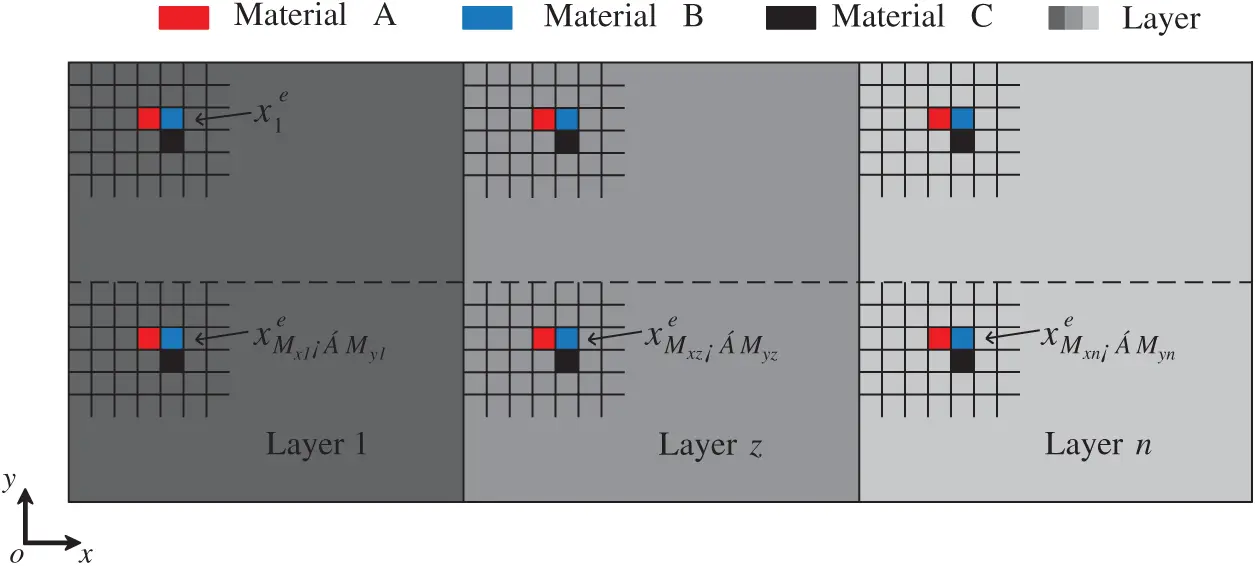Figure 5: Periodic layer-wise design

6  Numerical Examples

This paper proposes three numerical examples to illustrate the difference between the optimal topologies under loading uncertainties and deterministic loading conditions. For comparison, the settings in the DTO are the same as those in the RTO examples. In the calculation examples, the design domain is discretized by square elements, and the weight coefficients of mean value and standard deviation are set to 0.5, respectively. In order to prove the effectiveness of the proposed method, gradient layers of 2, 3, 4 and 6 are preset for different design domains in this paper, and for more intuitive comparison of DTO and RTO, 2×2, 2×1 and 1×1 are chosen for the sub-regions. Furthermore, the associated load amplitude and angle as well as the corresponding weights are calculated by Clenshaw-Curtis sparse grid.

6.1 Double-Sided Fixed Beam

The double-sided fixed beam is shown in Fig. 6. The design domain is a 160×80 plane quadrilateral structure, with both sides fixed, and the central position is subjected to the vertical downward concentrated load F, which is set as 1.0. For deterministic load conditions, the amplitude is set to 1, while load uncertainty, the amplitude and angle are independent random variables. The angle θ satisfied a continuous and uniform distribution with intervals of [-3π/4, -π/4] and the amplitude satisfied normal distribution, with the mean and standard deviation of 1 and 0.3, respectively. The allowable volume of the material is set to 20%. The material parameter settings are listed in Tab. 1.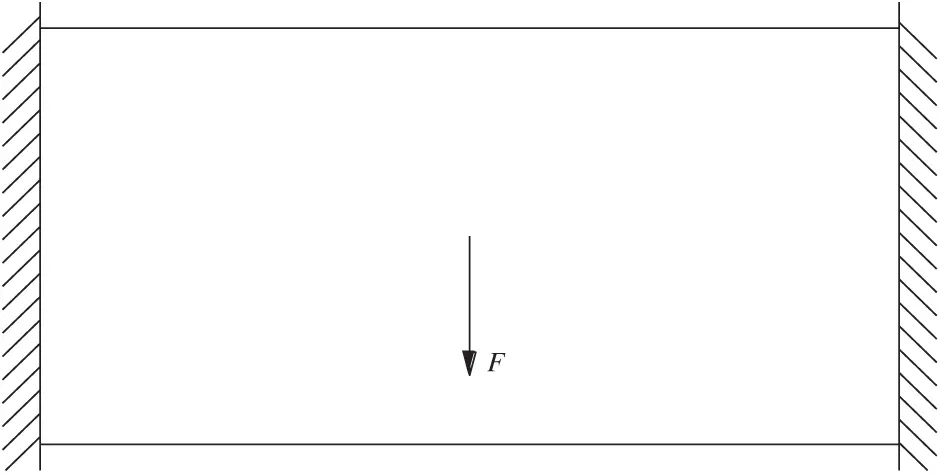Figure 6: Design domain of double-sided fixed beam structure

Table 1: Implementation of three materials parameters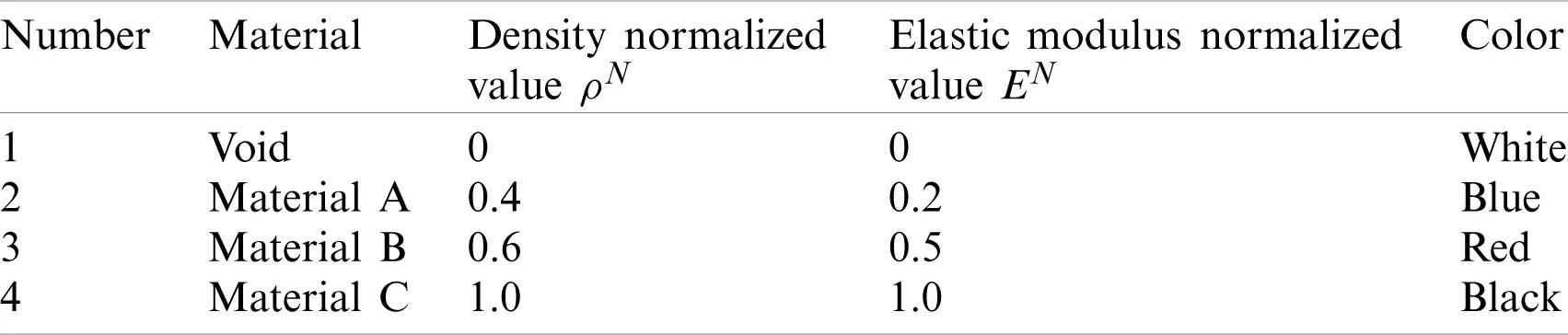The design domain is discretized into 12800 (160×80) square elements. Different materials combination schemes are considered as follows: (1) Scheme I: Material A, Material B, Material C and Void, (2) Scheme II: Material A, Material C and Void, (3) Scheme III: Material A, Material B and Void, (4) Scheme IV: Material A and Void. The gradient presets are applied to the design area along the X-axis, and Layer z = 2, 4. Each gradient layer is divided into 2×2, 2×1 and 1×1 sub-regions for discussion. The volume fraction of the preset gradient layer is obtained through the macroscopic topology optimization of deterministic design and robust design, as shown in Tab. 2. Then, the optimal periodic functionally graded topology material distribution of DTO and RTO is obtained, as exhibited in Figs. 7 and 8. The mean value, standard deviation and objective function of the Scheme II under Fig. 7 are summarized in Tab. 2.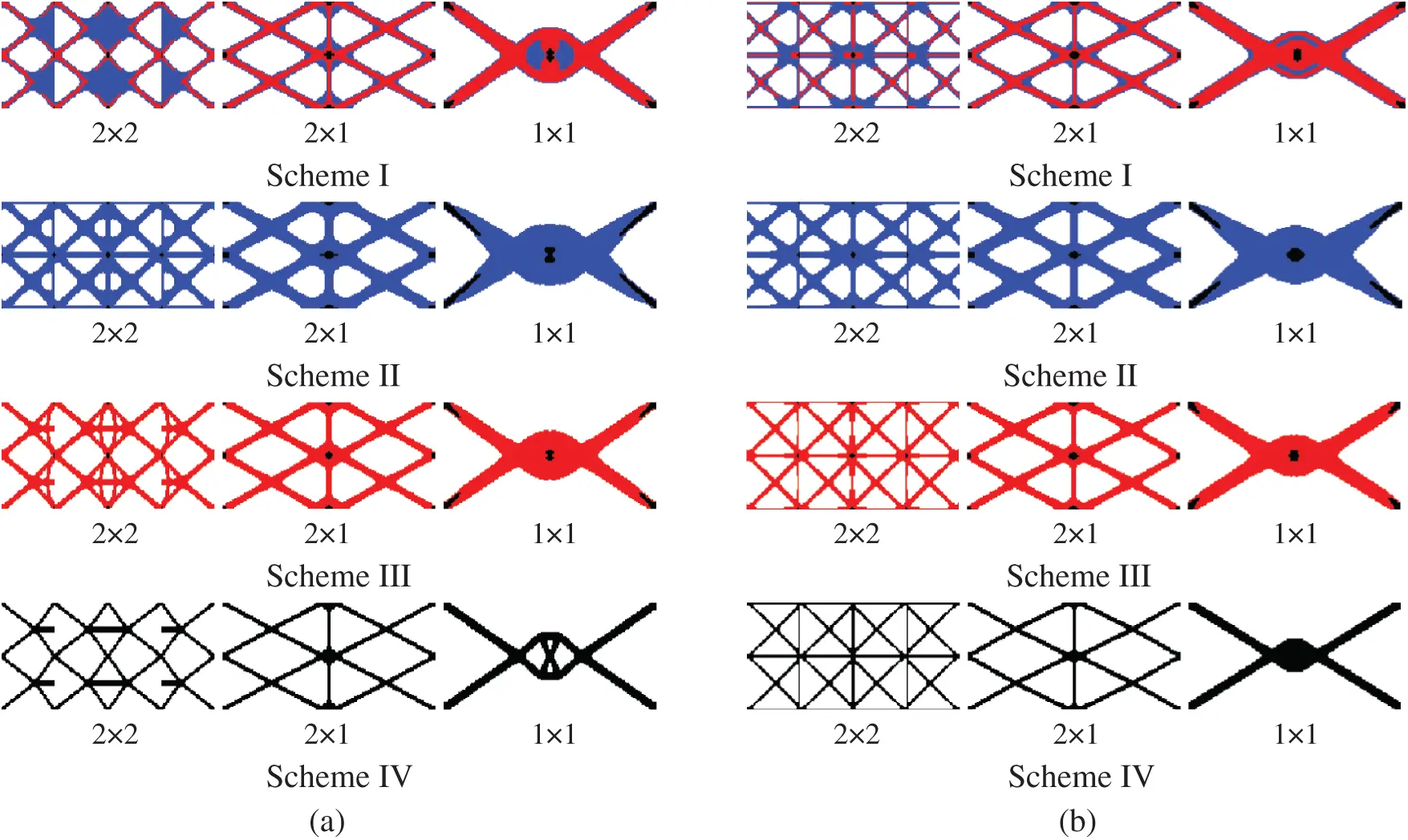Figure 7: Comparison of optimized topologies of multi-material DTO and RTO under two gradient layers. (a) Deterministic design (b) robust design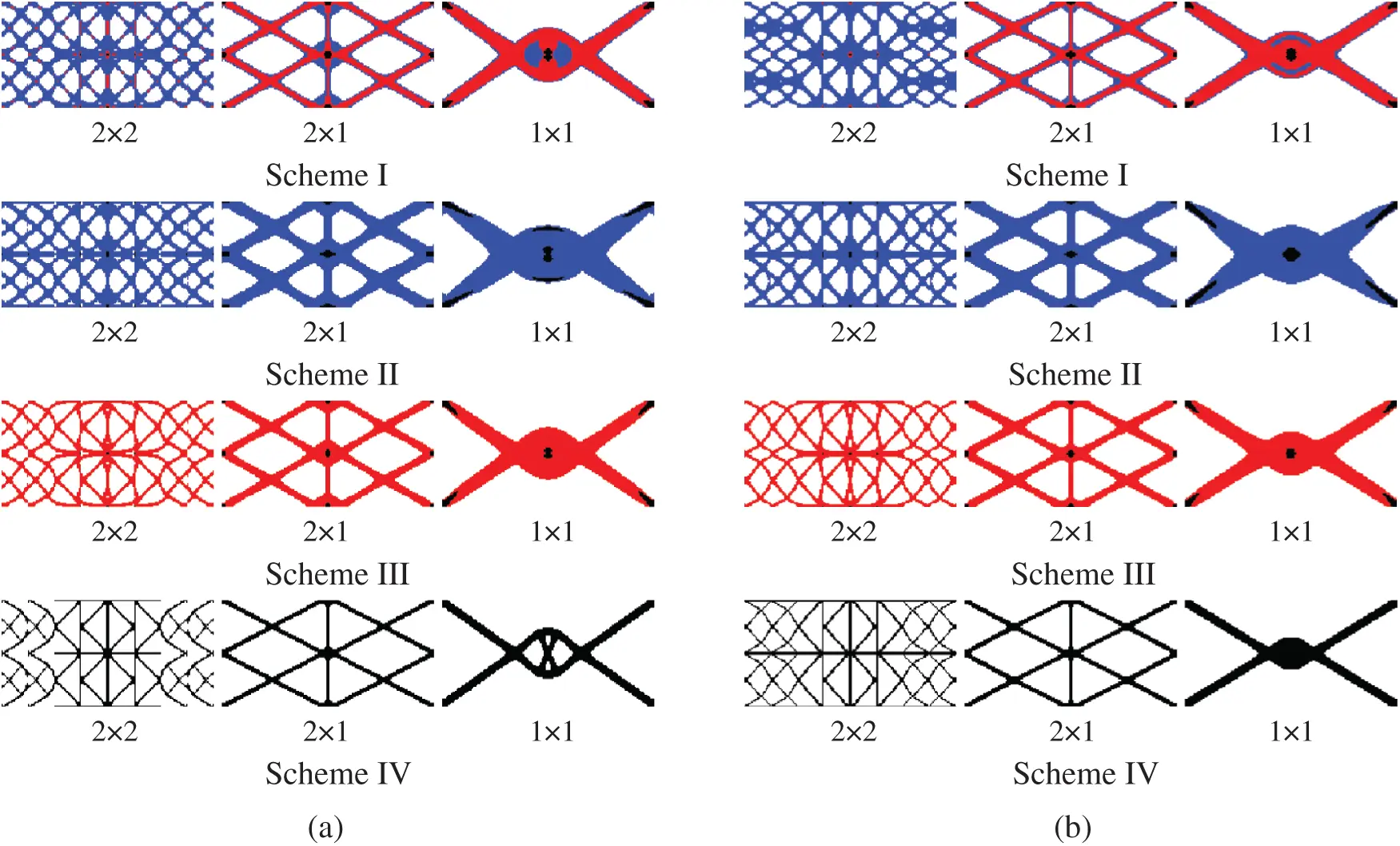Figure 8: Comparison of optimized topologies of multi-material DTO and RTO under four gradient layers. (a) Deterministic design (b) robust design

Table 2: Volume fraction of gradient layerThe optimization results show that the proposed method can effectively obtain the periodic functionally gradient structure under different gradient layers, periodic division and material combination schemes, and determine the reasonable material distribution, which shows the effectiveness of the proposed method. Compared with the DTO, the materials of the robust optimization structure are more concentrated on the horizontal force transmission route, thus improving the horizontal load capacity of the structure. It can be seen from Tab. 3 that the mean, standard deviation and objective function of the structural compliance obtained from the deterministic design are all higher than those obtained from the robust design. Therefore, the results of RTO have better stability under load uncertainty.

Table 3: The topology design result of double-sided fixed beamFor the periodic functionally graded structure under the layered setting, the optimal periodic structure of each gradient layer can be obtained. According to the 2×2 of scheme I under Figs. 7 and 8, substructure of gradient layer are shown in Tab. 4. With the increase of the number of gradient layers, the optimal configurations of the structure exhibit distinct topologies.

Table 4: Substructure of gradient layer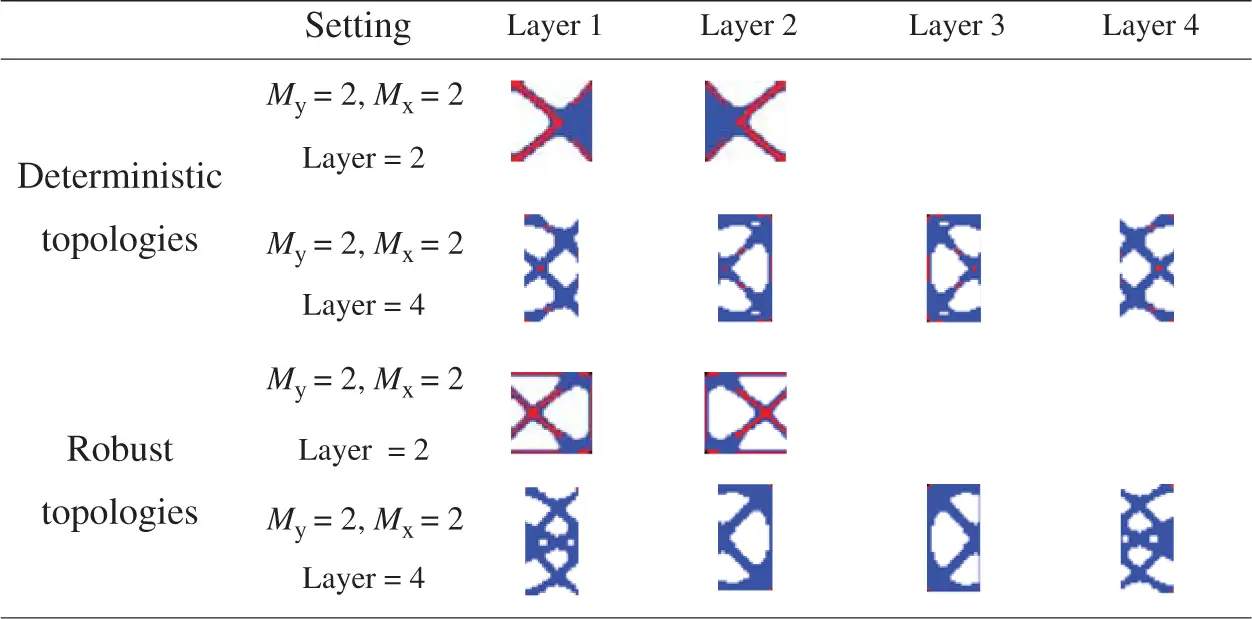6.2 Cantilever Beam

The design domain of cantilever beam is a 120×120 quadrilateral with the left edge fixed, as shown in Fig. 9. The upper right corner of the structure is subjected to a vertical upward uncertain load F1, and the lower right corner acted as a vertical downward uncertain load F2 that are assumed to be 1.0. The load condition and the parameters of material A and C are the same as those of Section 6.1. Let the normalized value of density ρN=0.7, and the normalized value of elastic modulus of material B EN = 0.8. The structural volume fraction is limited within 30%.Figure 9: Design domain for a cantilever beam

The design domain is discretized into 14400 (120×120) square elements. The gradient presets are applied to the design area along the X-axis, and Layer z = 3. Each gradient layer is divided into 2×2, 2×1 and 1×1 sub-regions for discussion. The optimal periodic functionally graded topology material distributions of deterministic design and robust design are exhibited in Fig. 10.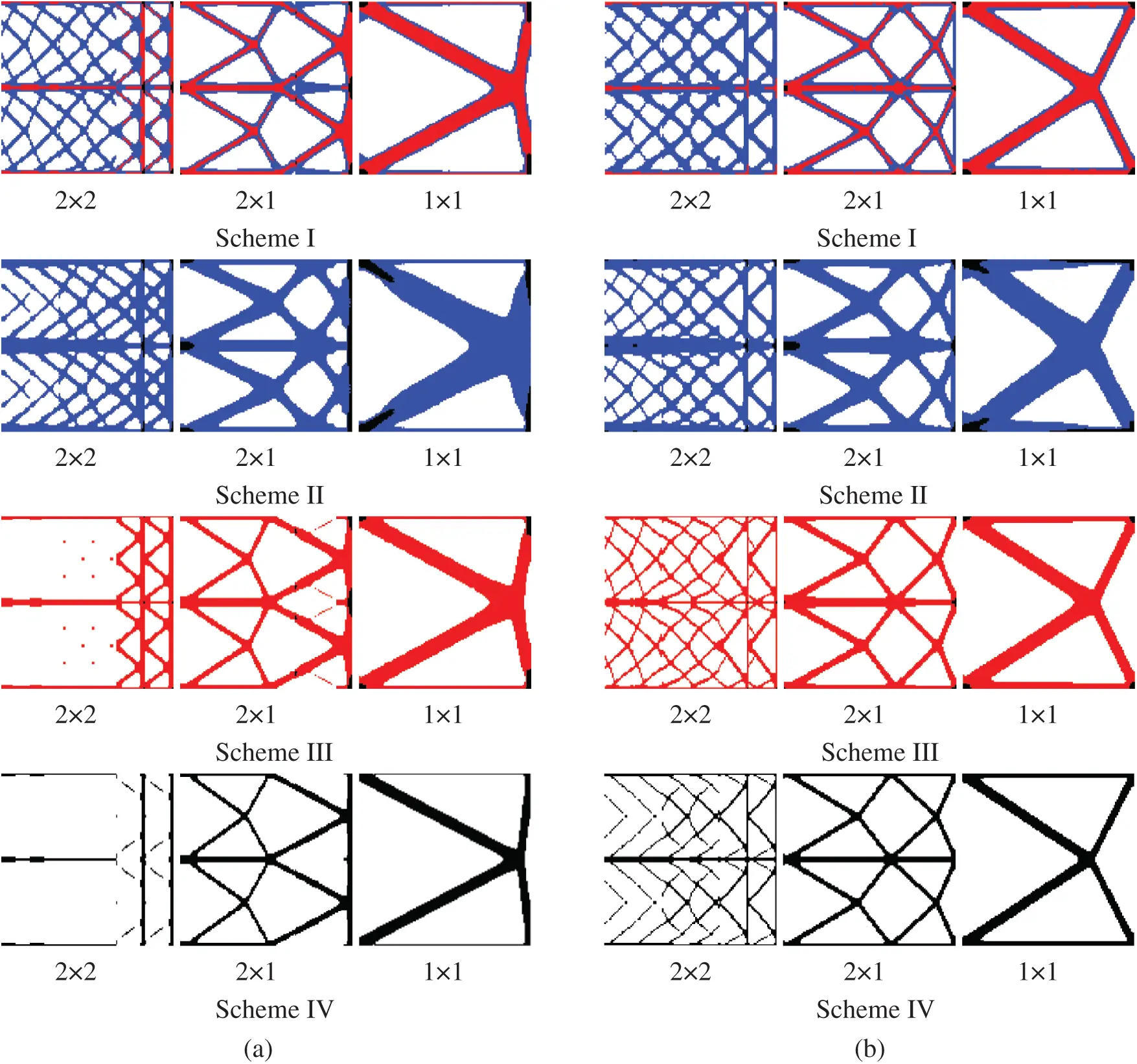Figure 10: Comparison of optimized topologies of multi-material DTO and RTO under three gradient layers. (a) Deterministic design (b) robust design

The optimization results show that the optimized structures are symmetrical because the design domain, boundary conditions, and load effects of the two working conditions are all symmetrical. Compared with the deterministic design, the optimized results of the robust design have thicker upper and lower edges and better horizontal load bearing capacity due to the uncertain loads that generates horizontal partitioning. Although such a structure has a reduced vertical load bearing capacity, the horizontal load bearing capacity is enhanced and the overall structure becomes more stable.

Meanwhile, considering the influence of periodic functional gradient constraints, we observed that the material distribution of the fixed edge of the DTO was not obvious, and the load bearing capacity was weak. On the contrary, the overall material distribution of the RTO results is reasonable, which further proves that it exhibits better stability.

The convergence process is rapid and stable, indicating the stability of the algorithm. According to the 2×1 of Scheme I under Fig. 10, the convergence curve of the topology optimization process of structural deterministic design and robust design is shown in Fig. 11. The horizontal axis represents the number of iterations, and the vertical axis represents the objective function. The mean value, standard deviation and objective function of the multi-material topology optimization design under Scheme I are summarized in Tab. 5. As can be seen from the table, the mean, standard deviation and objective function of the structural compliance obtained from the deterministic design are all higher than those obtained from the robust design, which proves the effectiveness of the algorithm.Figure 11: Variation curve of mean, standard deviation and objective function of compliance with the number of iterations. (a) Deterministic design (b) robust design

Table 5: The topology design result of cantilever beam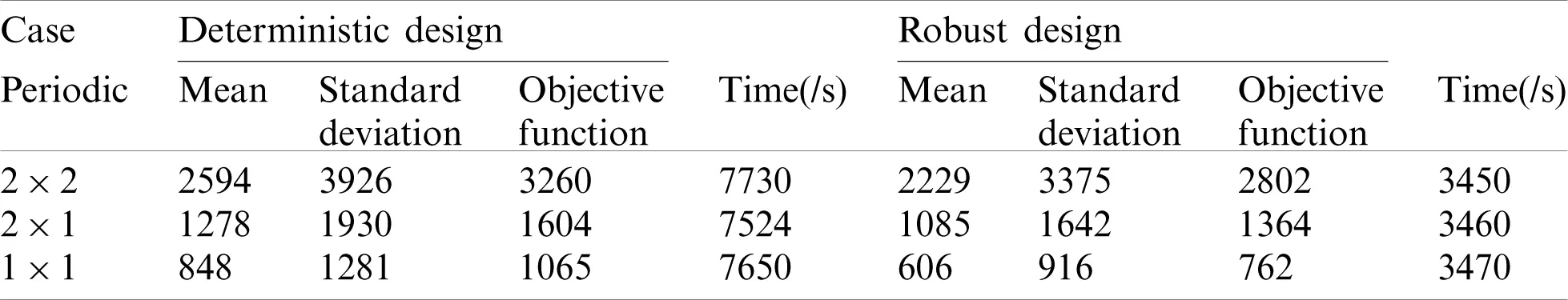6.3 Simply Supported Beam

The simply supported beam structure is shown in Fig. 12. The design domain is a 180×60 planar quadrilateral. Both edges of the bottom are simply supported, and uniform loads are applied to the top. The other settings are the same as in Section 6.1. The random field covariance relationship is as follows:

C= exp(-X1-X2d)(33)

where X1-X2 is the Euclidean distance between two nodes, and d is the correlation length set to 60.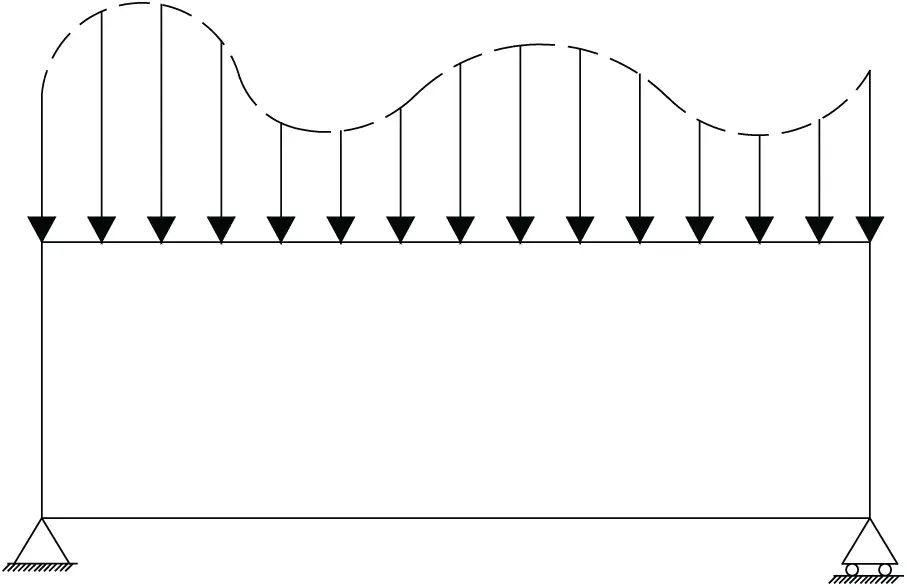Figure 12: Simple beam design space

The design domain is discretized into 10800 (180×60) square elements, and volume constraint of the material is set to 0.2. The material density and elastic modulus are the same as those in Section 6.1. Let the filtering radius Rmin=3, and the weight coefficient of mean and standard deviation be 0.5. The gradient presets are applied to the design area along the X-axis, and Layer z = 3, 6. Each gradient layer is divided into 2×2, 2×1 and 1×1 sub-regions for discussion. For the load condition random field, the K-L expansion is used to discretize into three random variables, which are denoted as

F(θ)=μF+i=13λiφiμi(θ)(34)

where μF represents the average load. According to the sparse grid numerical integration method, the results of deterministic topology optimization design and robust topology optimization design are exhibited in Figs. 13 and 14, respectively.Figure 13: Comparison of optimized topologies of multi-material DTO and RTO under three gradient layers. (a) Deterministic design (b) robust design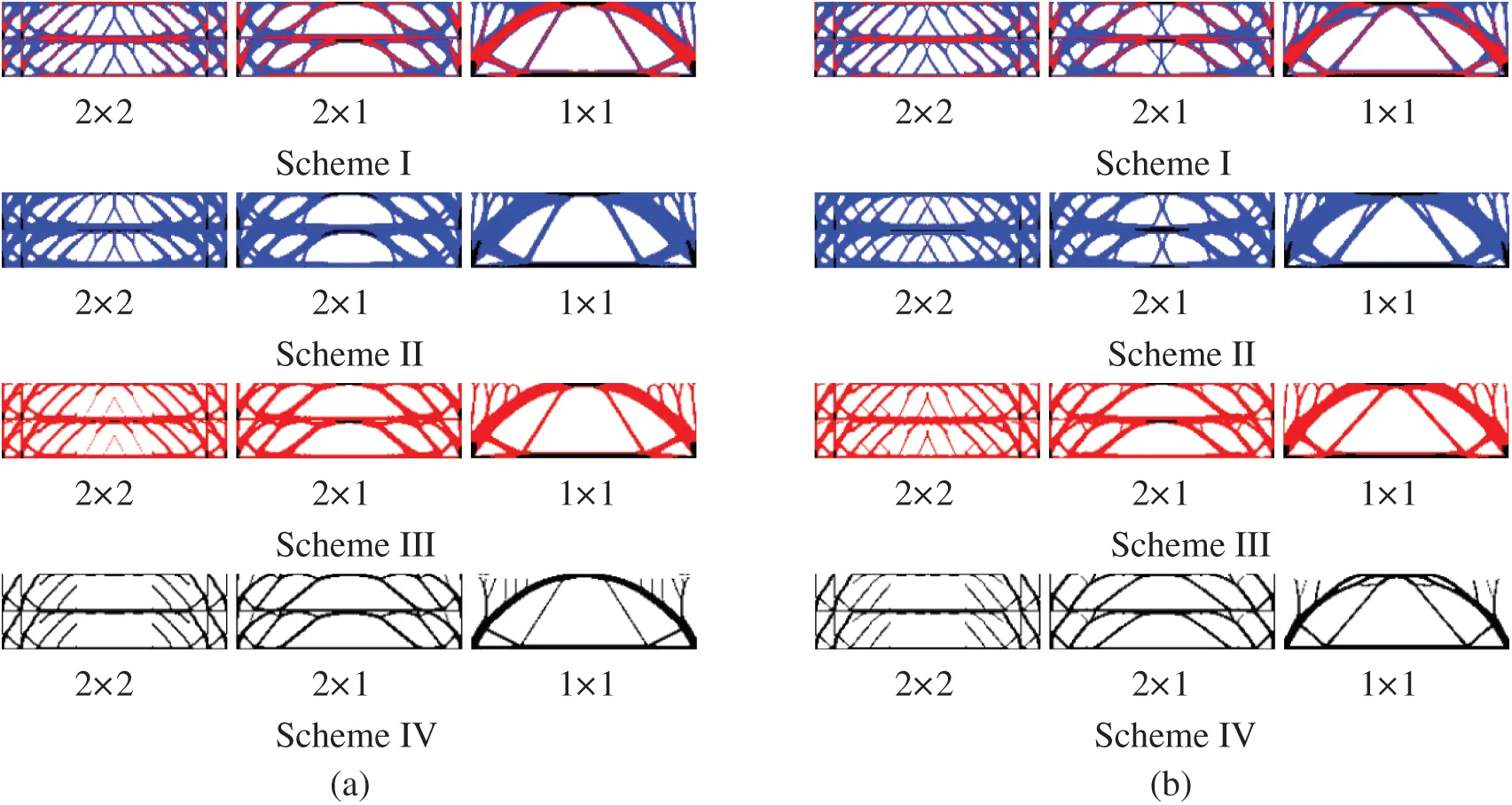Figure 14: Comparison of optimized topologies of multi-material DTO and RTO under six gradient layers. (a) Deterministic design (b) robust design

It can be seen from the Figs. 13 and 14 that the boundary of the optimization result is clear, and that the periodic setting in the gradient layer clearly demonstrates the effectiveness of this method. Due to the superposition of multiple loads under the influence of different amplitudes, the configuration change of RTO was significantly different from that of DTO, which was mainly reflected in the obvious supporting effect of filling the rod-shaped materials in the middle of the structure, thus improving the stability of the structure. Meanwhile, the model has excellent applicability to different material combination schemes.

According to the 2×2 of Scheme I under Figs. 13 and 14, the deterministic optimization results and robust optimization results are shown in Tab. 6. It can be seen from the table that the compliance mean, standard deviation, and objective function of the RTO are all lower than those of the DTO. Therefore, the robust design structure is more robust and stable. Fig. 15 shows the curve of the objective function with the number of iteration steps under different gradient layer 2×2 period settings. As shown in the figure, the objective function converges rapidly and smoothly, which further proves the stability of the proposed method.

Table 6: The topology design result of simply supported beam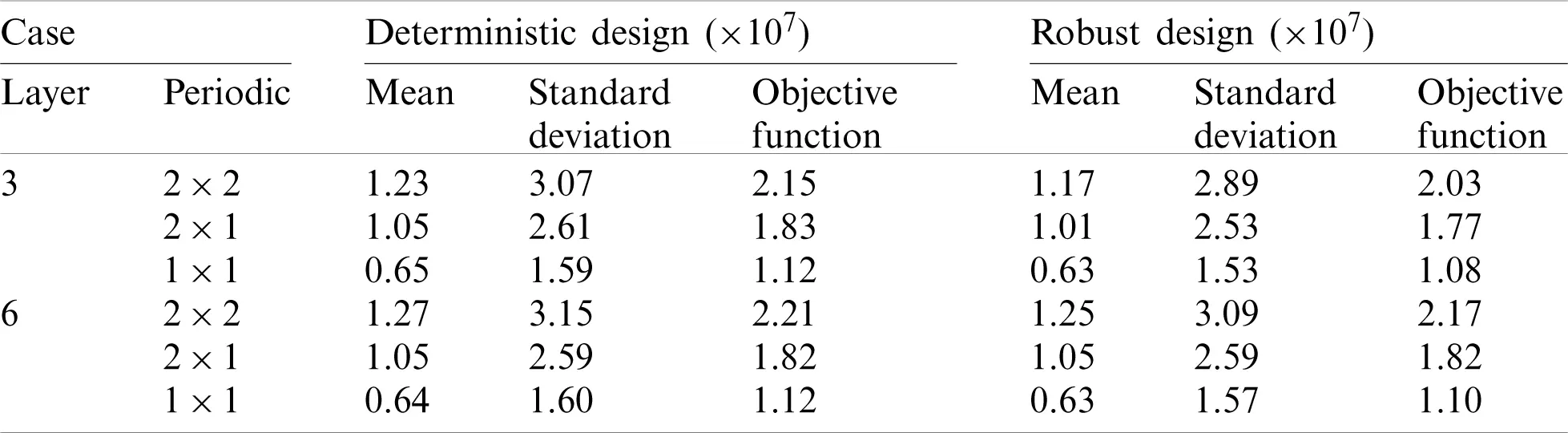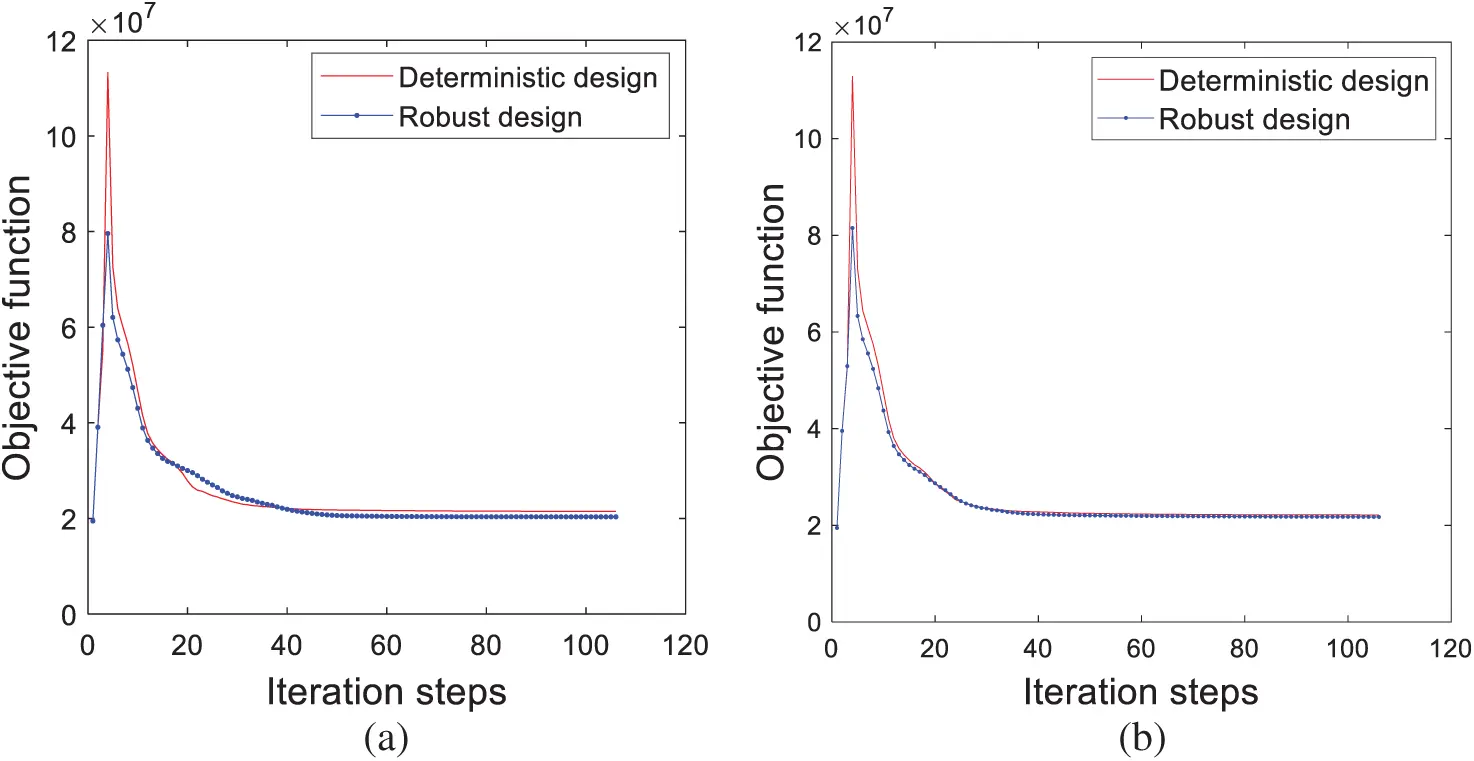Figure 15: Variation curve of objective function of compliance with the number of iterations. (a) Three layer gradient, (b) six layer gradient

7  Conclusions

This paper presents a robust topology optimization design method for multi-material functional gradients considering periodic constraints. In order to optimize the topology of the multi-material functionally graded structure and minimize the structural compliance under the volume constraint, an ordered-SIMP interpolation is proposed. Meanwhile, the structure is set periodically considering the practical engineering applications. Then, the sparse grid numerical integration method is introduced to calculate the objective function, the mean and standard deviation solutions for the robust topological optimization design. The design is explored in terms of both deterministic and robust design for different load conditions. By three arithmetic examples, the effectiveness of the design method is demonstrated. From the results, it can be seen that the robust design provides a more reliable and effective design solution compared with the corresponding deterministic topology optimization design. In addition, the proposed approach yields better design results for different functional gradient settings and material combination schemes, further demonstrating the practicality of the design solution.

Acknowledgement: The authors are thankful for Professor Krister Svanberg for MMA program made freely available for research purposes and the anonymous reviewers for their helpful and constructive comments.

Funding Statement: This work is supported by the Natural Science Foundation of China (Grant 51705268) and China Postdoctoral Science Foundation Funded Project (Grant 2017M612191).

Conflicts of Interest: The authors declare that we have no conflicts of interest to report regarding the present study.

## References

1. Birman, V. (2007). Modeling and analysis of functionally graded materials and structures. Applied Mechanics Reviews, 60(5), 42. [Google Scholar] [CrossRef]
2. Li, H., Luo, Z., Gao, L., & Walker, P. (2017). Topology optimization for functionally graded cellular composites with metamaterials by level sets. Computer Methods in Applied Mechanics & Engineering, 328(1), 340-364. [Google Scholar] [CrossRef]
3. Paulino, G. H., & Silva, E. C. N. (2005). Design of functionally graded structures using topology optimization. Materials Science Forum, 492, 435-440. [Google Scholar]
4. Xia, Q., & Wang, M. Y. (2008). Simultaneous optimization of the material properties and the topology of functionally graded structures. Computer Aided Design, 40(6), 660-675. [Google Scholar] [CrossRef]
5. Huang, X. D., Zhou, S. W., Xie, Y. M., & Li, Q. (2013). Topology optimization of microstructures of cellular materials and composites for macrostructures. Computational Materials Science, 67(2), 397-407. [Google Scholar] [CrossRef]
6. Li, H., Gao, L., Li, H., & Tong, H. (2020). Spatial-varying multi-phase infill design using density-based topology optimization. Computer Methods in Applied Mechanics and Engineering, 372, 113354. [Google Scholar] [CrossRef]
7. Guo, X., Bai, W., Zhang, W., & Gao, X. X. (2009). Confidence structural robust design and optimization under stiffness and load uncertainties. Computer Methods in Applied Mechanics and Engineering, 198(41), 3378-3399. [Google Scholar] [CrossRef]
8. Chen, S., Chen, W., & Lee, S. (2010). Level set based robust shape and topology optimization under random field uncertainties. Structural Multidisciplinary Optimization, 41(4), 507-524. [Google Scholar] [CrossRef]
9. Dunning, P. D., Kim, H. A., & Mullineux, G. (2011). Introducing loading uncertainty in topology optimization. AIAA Journal, 49(4), 760-768. [Google Scholar] [CrossRef]
10. Carrasco, M., Ivorra, B., & Ramos, A. M. (2012). A variance-expected compliance model for structural optimization. Journal of Optimization Theory and Applications, 152(1), 136-151. [Google Scholar] [CrossRef]
11. Lazarov, B. S., Schevenels, M., & Sigmund, O. (2012). Topology optimization with geometric uncertainties by perturbation techniques. International Journal for Numerical Methods in Engineering, 90(11), 1321-1336. [Google Scholar] [CrossRef]
12. Guo, X., Zhang, W., & Zhang, L. (2013). Robust structural topology optimization considering boundary uncertainties. Computer Methods in Applied Mechanics and Engineering, 253, 356-368. [Google Scholar] [CrossRef]
13. Guest, J. K., & Igusa, T. (2008). Structural optimization under uncertain loads and nodal locations. Computer Methods in Applied Mechanics and Engineering, 198(1), 116-124. [Google Scholar] [CrossRef]
14. Dunning, P. D., Kim, H. A., & Mullineux, G. (2011). Introducing loading uncertainty in topology optimization. AIAA Journal, 49(4), 760-768. [Google Scholar] [CrossRef]
15. Kharmanda, G., Olhoff, N., Mohamed, A., & Lemaire, M. (2004). Reliability-based topology optimization. Structural & Multidisciplinary Optimization, 26(5), 295-307. [Google Scholar] [CrossRef]
16. Knoll, P., & Reich, S. (2010). Level set based robust shape and topology optimization under random field uncertainties. Structural & Multidisciplinary Optimization, 41(4), 507-524. [Google Scholar] [CrossRef]
17. Zhao, Q. H., Chen, X. K., Ma, Z. D., & Lin, Y. (2015). Robust topology optimization based on stochastic collocation methods under loading uncertainties. Mathematical Problems in Engineering, 580980(1), 1-14. [Google Scholar] [CrossRef]
18. Long, K., Wang, X., & Du, Y. X. (2018). Robust topology optimization formulation including local failure and load uncertainty using sequential quadratic programming. International Journal of Mechanics and Materials in Design, 15(2), 317-332. [Google Scholar] [CrossRef]
19. Ben-Tal, A., & Nemirovski, A. (1997). Robust truss topology design via semidefinite programming. SIAM Journal on Optimization, 7(4), 991-1016. [Google Scholar] [CrossRef]
20. Amir, O., Sigmund, O., Lazarov, B. S., & Schevenels, M. (2012). Efficient reanalysis techniques for robust topology optimization. Computer Methods in Applied Mechanics and Engineering, 245(33–34), 217-231. [Google Scholar] [CrossRef]
21. Wu, J., Gao, J., Luo, Z., & Brown, T. (2016). Robust topology optimization for structures under interval uncertainty. Advances in Engineering Software, 99, 36-48. [Google Scholar] [CrossRef]
22. Zhang, X., He, J., Takezawa, A., & Kang, Z. (2018). Robust topology optimization of phononic crystals with random field uncertainty. International Journal for Numerical Methods in Engineering, 115(9), 1154-1173. [Google Scholar] [CrossRef]
23. Kang, Z., Wu, C., Luo, Y., & Li, M. (2018). Robust topology optimization of multi-material structures considering uncertain graded interface. Composite Structures, 208, 305-406. [Google Scholar]
24. Chan, Y. C., Shintani, K., & Chen, W. (2019). Robust topology optimization of multi-material lattice structures under material and load uncertainties. Frontiers of Mechanical Engineering, 14(2), 141-152. [Google Scholar] [CrossRef]
25. Zheng, Y., Da, D., Li, H., Xiao, M., & Gao, L. (2020). Robust topology optimization for multi-material structures under interval uncertainty. Applied Mathematical Modelling, 78(5), 627-647. [Google Scholar] [CrossRef]
26. Romero, A. A., & Giusti, S. M. (2020). A robust topological derivative-based multi-material optimization approach: Optimality condition and computational algorithm. Computer Methods in Applied Mechanics and Engineering, 366(1), 113044. [Google Scholar] [CrossRef]
27. Chen, Z., Long, K., Wen, P., & Nouman, S. (2020). Fatigue-resistance topology optimization of continuum structure by penalizing the cumulative fatigue damage. Advances in Engineering Software, 150(2), 102924. [Google Scholar] [CrossRef]
28. Zheng, W., Wang, Y. J., Zheng, Y. F., & Da, D. (2020). Efficient topology optimization based on DOF reduction and convergence acceleration methods. Advances in Engineering Software, 149, 102890. [Google Scholar] [CrossRef]
29. Wang, Y. J., Liao, Z. Y., Ye, M., Zhang, Y., & Li, W. H. (2020). An efficient isogeometric topology optimization using multilevel mesh, MGCG and local-update strategy. Advances in Engineering Software, 139(8), 102733. [Google Scholar] [CrossRef]
30. Zuo, W. J., & Saitou, K. (2017). Multi-material topology optimization using ordered SIMP interpolation. Structural & Multidisciplinary Optimization, 55(2), 477-491. [Google Scholar] [CrossRef]
31. Li, C. C., & Kiureghian, A. D. (1993). Optimal discretization of random fields. Journal of Engineering Mechanics, 119(6), 1136-1154. [Google Scholar] [CrossRef]
32. Ghanem, R., Spanos, P. (2003). Stochastic finite elements—A spectral approach. New York: Dover.
33. Xiu, D. (2010). Numerical methods for stochastic computations: A spectral method approach (Ph.D. Thesis). Princeton University Press, Princeton.
34. Ghanem, R. G., Spanos, P. D. (1991). Stochastic finite elements: A spectral approach. New York: Springer.
35. Kang, Z., & Liu, P. (2018). Reliability-based topology optimization against geometric imperfections with random threshold model. International Journal for Numerical Methods in Engineering, 115(1), 99-116. [Google Scholar] [CrossRef]
36. Haldar, A., Mahadevan, S. (2000). Reliability assessment using stochastic finite element analysis. New York: Wiley.
37. Ghanem, R. G., & Doostan, A. (2006). On the construction and analysis of stochastic models: Characterization and propagation of the errors associated with limited data. Journal of Computational Physics, 217(1), 63-81. [Google Scholar] [CrossRef]
38. Beyer, H., & Sendhoff, B. (2007). Robust optimization: A comprehensive survey. Computer Methods in Applied Mechanics and Engineering, 196(33–34), 3190-3218. [Google Scholar] [CrossRef]
39. Park, S. H. (1996). Robust design and analysis for quality engineering. London: Chapman and Hall.
40. Meng, Z., Wu, Y., Wang, X., Ren, S., & Yu, B. (2020). Robust topology optimization methodology for continuum structures under probabilistic and fuzzy uncertainties. International Journal for Numerical Methods in Engineering, 1, 1-17. [Google Scholar]
41. Gao, J., Luo, Z., Li, H., & Gao, L. (2019). Topology optimization for multiscale design of porous composites with multi-domain microstructures. Computer Methods in Applied Mechanics and Engineering, 334, 451-476. [Google Scholar] [CrossRef]
42. Gao, J., Luo, Z., Li, H., & Gao, L. (2019). Dynamic multiscale topology optimization for multi-regional micro-structured cellular composites. Composite Structures, 211, 401-417. [Google Scholar] [CrossRef]
43. Eldred, M. S., Webster, C. G., & Constantine, P. G. (2011). Design under uncertainty employing stochastic expansion methods. International Journal for Uncertainty Quantification, 1(2), 119-146. [Google Scholar] [CrossRef]
44. Aicke, H., Erich, N., & Mario, U. (2014). On weak tractability of the clenshaw–curtis smolyak algorithm. Journal of Approximation Theory, 183, 31-44. [Google Scholar] [CrossRef]
45. Svanberg, K. (2010). The method of moving asymptotes—A new method for structural optimization. International Journal for Numerical Methods in Engineering, 24(2), 359-373. [Google Scholar] [CrossRef]This work is licensed under a Creative Commons Attribution 4.0 International License, which permits unrestricted use, distribution, and reproduction in any medium, provided the original work is properly cited.